# We Helped With This Electrical Engineering Assignment: Have A Similar One?

SOLVEDCategory Engineering Electrical Engineering Graduate Solved Electrical Engineering Homework Help

## Short Assignment Requirements

The goal of this first assignment is to use Matlab/Simulink to simulate the plant (open loop) of each application. The submissions should include the picture of the diagram via Simulink simulation and the response to initial conditions and/or to a unit step input. And then comment on whether the transient response observed on the scope is reasonable.It is just #1 for each case study for the first assignment due on the 28th. Total of 4 problems.

## Assignment Description

Application Studies for Control System Design

1/21/13

### DCS – DC Servomotor

Dynamics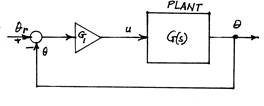θ˙ = ω

ω˙ = −αω + βu y = θ

Let

x1 = y,        x2 = ω,       α = 4,        β = 1

Application studies

1.    Simulate open-loop system

2.    Calculate plant transfer function G(s) = C(sI A)−1B

3.    Closed-loop proportional control: u = −G1(θ θr) (See block diagram above.)

(a)    Analytically show that closed-loop system is stable for all values of G1.

(b)    Obtain Nyquist plot using Matlab.

(c)     Obtain Bode plot using Matlab.

(d)    Sketch Root locus and verify using Matlab.

(e)    Develop closed-loop simulation and simulate performance for several values of G1.

### PEN – Motor-Driven Pendulum

Dynamics

θ˙ = ω

ω˙ = −αω + (g/L)sinθ + βu y = θ

Let

x1 = y,        x2 = ω,       α = 4,        β = 1,      g/L = 12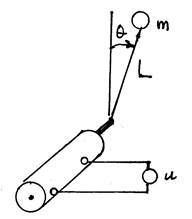Application studies

1.    Simulate open-loop system

2.    Obtain the plant transfer functions G(s) = C(sI A)−1B for

θ = 0

θ = π

Do this analytically and using the Matlab function linmod

3.    Closed-loop proportional control for θ = π and u = −G1(θ θr)

(a)    Analytically show that closed-loop system is stable for all values of G1.

(b)    Obtain Nyquist plot using Matlab

(c)     Obtain Bode plot using Matlab

(d)    Sketch Root locus and verify using Matlab.

(e)    Develop closed-loop simulation and simulate performance for several values of G1.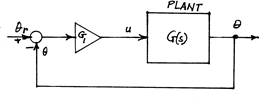4.    Closed-loop proportional control for θ = 0 and u = −G1(θ θr)

(a)    Find the range of G1 for which the system is stable.

(b)    Obtain Nyquist plot.

(c)     Obtain Bode plot.

(d)    Sketch Root locus and verify using Matlab.

(e)    Develop closed-loop simulation and simulate performance for several values of G1.

5.    Full-state feedback, θr = 0, Pole placement For each equilibrium state (θ = 0= π determine the full state feedback gains that place the closed-loop poles at

(a)    s = −6 ± 4j

(b)    s = −8,−10

For each equilibrium state and set of control gains, simulate the closed loop behavior, and comment on the results.

6.    Full-state feedback with reference input. For θr = 0.5 compute the gain G0 for the exogenous input for each equilibrium state in Part 5, and simulate the performance. Verify that the steady-state error goes to zero.

### TH3 – Third-Order Heat Conduction

Dynamics

x˙1 = −3x1 + x2 + u x˙2 = x1− 2x2x3 x˙3 = x2− 3x3 + x0 y = x3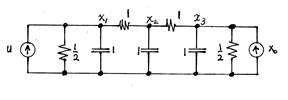Application Studies

1.    Simulate open-loop system

2.    Calculate plant transfer function G(s) = C(sI A)−1B

3.    Closed-loop proportional control: u = −G3(x3xr)

(a)    Find the range of G3 for which the closed-loop system is stable.

(b)   Obtain Nyquist plot using Matlab(c) Obtain Bode plot using Matlab.

(d)   Sketch Root locus and verify using Matlab.

(e)    Develop a closed-loop simulation and simulate performance for several values of G3.

(f)     Determine the steady-state error for a unit step reference input as a function of G3. 4. Closed-loop proportional + integral control For the closed-loop control law

U(s) = −(Gp + GI/s)(Y (s) − Yr(s))

(a)    Show that the steady state error is zero for GI > 0

(b)    Find the region in the Gp,GI plane for which the system is stable.

(c)     Develop a closed-loop simulation and simulate performance for several values of GP,GI within the range of stability.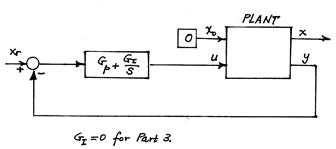5.    Design a full-state feedback control law that places the closed-loop poles at s = −10 ± 5j,−15 and accounts for a non-zero nonzero reference value, and for a nonzero exogenous constant input. Simulate closed-loop performance.

6.    Design a full-order observer for x0 = 0 ( and for y = x3) with poles at s = −20 + 10j,−30. Using this observer with the gain matrix of Part 5, design the full-order compensator and simulate performance

7.    Augment the observer of Part 6 by the additional state variable to represent the reference and exogenous inputs.

8.    Design (and simulate performance) of a compensator using a reduced-order observer, with poles ats = −20 ± 10j

9.    Design (and simulate performance) of a compensator with gains designed for a linear-quadratic regulator with

Q = diag[0,0,q3],        R = 1

and a full-order Kalman filter using

F = B, V = v,, W = 1

Choose q3 and v to make the step response five times faster than open-loop with overshoot less than 5% . This is to be done by simulation.

### PCA – Pendulum on Cart

Linearized Dynamics

For an inverted pendulum on a cart, driven by a d-c motor, the linearized dynamics are

x¨ = −αx˙ − (mg/M)θ + βu

θ¨ = (α/L)x˙ + ((M + m)g/ML)θ − (β/L)u

The state and output variables are

x1 = x, x2 = θ, x3 = x, x˙          4 = θ, y˙      1 = x1, y2 = x2

Numerical values of the parameters are:

α = 4, β = 1, L = 0.25M, m = 0.4Kg, M = 1Kg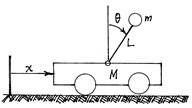### Application Studies

1.        Develop a simulation of the system

2.        Calculate the transfer functions from the input u to the outputs y1 and y2. (Do this analytically–with the aid of Matlab’s Symbolic Toolbox, if necessary, and verify using the Control System Toolbox.)

3.        Find the open loop poles and the zeros of each transfer function.

4.        Determine whether the system can be stabilized by the control law

u = −G1x1

5.        Determine whether the system can be stabilized by the control law

u = −G1x1G2x2

6.        Determine the full state feedback gains that place the closed-loop poles at

(a)    s = −10 ± 5j,−20 ± 10j

(b)    s = −10,−15,−20,−30

Simulate the transient response using this gain matrix. (Investigate whether the poles farther from the origin are very important.)

(a)    For an initial position offset (x1 = .1), all other state variables initially zero, for both set of gains.

(b)    For an initial angular error (x2 = .1), all other state variables zero, for both sets of gains.

7.        Design a full-order observer having poles at s = −40 ± 30j,−60 ± 20j. Simulate the performance with this observer in place and using the gain matrix determined in Part 6. Compare the actual state and the state estimate produced by the observer.

8.        Design a reduced-order observer with poles at s = −40 ± 30j. Simulate the performance with this observer in place and using the gain matrix determined in Part 6. Compare the actual state and the state estimate produced by the observer.

9.        Design a full-state feedback control law using quadratic optimization (LQR) with matrices given by

Q = diag[q1,q2,0,0],        R = 1

Choose q1 and q2 to meet the following design goal (if possible). For an initial cart position error of

.1 m, and with the pendulum vertical,

Reduce the position error to zero in about 2 sec with overshoot <.01 m

Keep the pendulum angle < .05 rad.

The values of q1 and q2 are to be determined by simulation.

10.    Design a compensator by use of the gains obtained in Part 9 and the reduced-order observer of Part 8,and simulate performance.

11.    Determine the gains of a Kalman-filter based full-order observer. Use F = B and W = I2 (2 by 2 identity matrix). To pick the value of V , try to match the pole locations of Part 6.

Is it free to get my assignment evaluated?

Yes. No hidden fees. You pay for the solution only, and all the explanations about how to run it are included in the price. It takes up to 24 hours to get a quote from an expert. In some cases, we can help you faster if an expert is available, but you should always order in advance to avoid the risks. You can place a new order here.

How much does it cost?

The cost depends on many factors: how far away the deadline is, how hard/big the task is, if it is code only or a report, etc. We try to give rough estimates here, but it is just for orientation (in USD):

 Regular homework \$20 - \$150 Advanced homework \$100 - \$300 Group project or a report \$200 - \$500 Mid-term or final project \$200 - \$800 Live exam help \$100 - \$300 Full thesis \$1000 - \$3000

How do I pay?

Credit card or PayPal. You don't need to create/have a Payal account in order to pay by a credit card. Paypal offers you "buyer's protection" in case of any issues.

Why do I need to pay in advance?

We have no way to request money after we send you the solution. PayPal works as a middleman, which protects you in case of any disputes, so you should feel safe paying using PayPal.

Do you do essays?

No, unless it is a data analysis essay or report. This is because essays are very personal and it is easy to see when they are written by another person. This is not the case with math and programming.

Why there are no discounts?

It is because we don't want to lie - in such services no discount can be set in advance because we set the price knowing that there is a discount. For example, if we wanted to ask for \$100, we could tell that the price is \$200 and because you are special, we can do a 50% discount. It is the way all scam websites operate. We set honest prices instead, so there is no need for fake discounts.

Do you do live tutoring?

No, it is simply not how we operate. How often do you meet a great programmer who is also a great speaker? Rarely. It is why we encourage our experts to write down explanations instead of having a live call. It is often enough to get you started - analyzing and running the solutions is a big part of learning.

What happens if I am not satisfied with the solution?

Another expert will review the task, and if your claim is reasonable - we refund the payment and often block the freelancer from our platform. Because we are so harsh with our experts - the ones working with us are very trustworthy to deliver high-quality assignment solutions on time.

Customer Feedback

"Thanks for explanations after the assignment was already completed... Emily is such a nice tutor! "

Order #13073